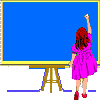Number, Algebra, Measures

has an appreciation of mathematical pattern and structure

has the ability to apply mathematical knowledge and skills to problem solving

is beginning to attempt mathematical tasks of increasing length and complexity

can select materials appropriate to a task and works methodically

records and presents findings systematically in a variety of forms

is able to read, write and order numbers and understands place value

demonstrates a good knowledge of number bonds

understands the meaning of negative numbers

recognises and understands fractions and percentages

has a good understanding of decimal notation and can apply this to money and measurement

is able to interpret a calculator display and can approximate to a given number of decimal places

can make and test statements and predictions

is able to measure and draw angles accurately

is able to use imperial units and knows the metric equivalents

has a sound knowledge of area and volume

gives up too easily when faced with a difficulty

needs to look at different ways of solving problems

does not yet realise that there may be more than one way of solving a problem

experiences difficulty in seeing number patterns

must check work thoroughly as is prone to careless errors

must learn tables thoroughly

does not have a sound enough knowledge of place value

relies too heavily on calculator

is not making enough use of estimation and approximation in determining a rough answer

lacks confidence when approaching new concepts and must ask for help when necessary

Shape, Space, Data Handling

has a positive attitude to mathematics and displays good spatial skills

shows independence in thinking and in methods used

has enjoyed a wide variety of mathematical activities both in and beyond the classroom

has gained a deeper understanding of mathematics through enquiry and practical activity

shows a keen interest in practical work and performs well both individually and as a member of a group

is able to use technical language when discussing the solution to a problem

plans work methodically and records findings systematically

has shown an interest in the construction of 3-D shapes using a range of materials

is able to recognise and describe two and three dimensional shapes and objects

is able to sort shapes in different ways and provide reasons for sorting

is able to understand and use language relating to angles

shows an understanding of probability

can construct and interpret charts, graphs and diagrams accurately

collects, records and processes data competently

is showing an increasing confidence in the collection and analysis of data

is able to enter and access information in simple data bases

needs to be more accurate when measuring

estimates are often wildly inaccurate

does not fully understand units of measurement and confuses centimetres and metres

does not fully understand probability, often confusing such things as 'unlikely' and 'impossible'

needs to plan work more carefully

finds new concepts difficult and rarely asks for help

must be more willing to explore and discuss alternative solutions to problems